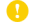Online ISSN : 1883-9029
Print ISSN : 0037-1114
ISSN-L : 0037-1114

ジャーナル フリー

1971 年 24 巻 3 号 p. 266-274[Correction] Zisin2 Vol.24 No.4 (1971) pp.e1f-e1f

There have been developed a number of relations between seismic wave energy E and the magnitude M of an earthquake. The general form of the relation is written as logE=α+βM. in which α, β are constants and M is the surface-wave magnitude. A number of relations were presented as given in Table 1 where α and β are listed. The relationship between α and β seems to keep linearity as shown in Fig. 1. This relation can therefore be expressed as α=(26.10±0.91)-(9.60±0.72)β for 5.3≤M≤8.5 (1) α=(19.11±0.70)-(4.59±0.40)β for -2.1≤M≤5.3 (2) An energy-magnitude relation for large earthquakes having magnitude larger than 5 seems to be different from that for small earthquakes having magnitude smaller than 5.
The lines expressing (1) and (2) intersect at the point α=12.66, β=1.40. Thus, a new magnitude-energy relation which is appropriate for both large and small earthquakes is obtained as logE=12.66+1.40M. By using the relations between α and β in (1) and (2), seismic efficiency factor f was obtained as 0.04-0.90 from some examples.著者関連情報